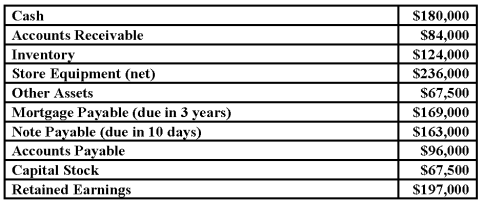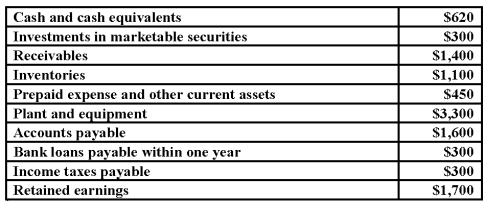/
/
/
118. Current ratio and working capitalThe balance sheet of Red Missile
Not my Question
Flag Content

# Question : 118. Current ratio and working capitalThe balance sheet of Red Missile : 1267477

118. Current ratio and working capital
The balance sheet of Red Missile Company contained the following items, among others:(a) From the above information compute:
(1) Current assets: \$_______
(2) Current liabilities: \$______
(3) The current ratio: ______ to 1
(4) Working capital: \$______
(b) Assume that Red Missile Company pays the note payable of \$163,000, thus reducing cash to \$17,000. Compute the following after the completion of this transaction:
(1) The current ratio: ______ to 1
(2) Working capital: \$______

119. Measures of solvency and credit risk
Shown below are selected items appearing in a recent balance sheet of Grant Products. (Dollar amounts are in thousands.)(a) Compute the following:
(1) Total quick assets \$____________
(2) Total current assets \$____________
(3) Total current liabilities \$____________
(4) Quick ratio ______ to 1
(5) Current ratio ______ to 1
(b) Research indicates an industry average quick ratio is 1.3 to 1, and a current ratio of 2.3 to 1. Based upon this information, does Grant Products appear more or less solvent than the average company in its industry? Explain briefly.

## Solution 5 (1 Ratings )

Solved
Accounting 3 Years Ago 55 Views